# 6.6: Simulating data for t-tests

$$\newcommand{\vecs}{\overset { \rightharpoonup} {\mathbf{#1}} }$$ $$\newcommand{\vecd}{\overset{-\!-\!\rightharpoonup}{\vphantom{a}\smash {#1}}}$$$$\newcommand{\id}{\mathrm{id}}$$ $$\newcommand{\Span}{\mathrm{span}}$$ $$\newcommand{\kernel}{\mathrm{null}\,}$$ $$\newcommand{\range}{\mathrm{range}\,}$$ $$\newcommand{\RealPart}{\mathrm{Re}}$$ $$\newcommand{\ImaginaryPart}{\mathrm{Im}}$$ $$\newcommand{\Argument}{\mathrm{Arg}}$$ $$\newcommand{\norm}{\| #1 \|}$$ $$\newcommand{\inner}{\langle #1, #2 \rangle}$$ $$\newcommand{\Span}{\mathrm{span}}$$ $$\newcommand{\id}{\mathrm{id}}$$ $$\newcommand{\Span}{\mathrm{span}}$$ $$\newcommand{\kernel}{\mathrm{null}\,}$$ $$\newcommand{\range}{\mathrm{range}\,}$$ $$\newcommand{\RealPart}{\mathrm{Re}}$$ $$\newcommand{\ImaginaryPart}{\mathrm{Im}}$$ $$\newcommand{\Argument}{\mathrm{Arg}}$$ $$\newcommand{\norm}{\| #1 \|}$$ $$\newcommand{\inner}{\langle #1, #2 \rangle}$$ $$\newcommand{\Span}{\mathrm{span}}$$$$\newcommand{\AA}{\unicode[.8,0]{x212B}}$$

An “advanced” topic for $$t$$-tests is the idea of using R to simulations for $$t$$-tests.

If you recall, $$t$$ is a property of a sample. We calculate $$t$$ from our sample. The $$t$$ distribution is the hypothetical behavior of our sample. That is, if we had taken thousands upon thousands of samples, and calculated $$t$$ for each one, and then looked at the distribution of those $$t$$’s, we would have the sampling distribution of $$t$$!

It can be very useful to get in the habit of using R to simulate data under certain conditions, to see how your sample data, and things like $$t$$ behave. Why is this useful? It mainly prepares you with some intuitions about how sampling error (random chance) can influence your results, given specific parameters of your design, such as sample-size, the size of the mean difference you expect to find in your data, and the amount of variation you might find. These methods can be used formally to conduct power-analyses. Or more informally for data sense.

## Simulating a one-sample t-test

Here are the steps you might follow to simulate data for a one sample $$t$$-test.

1. Make some assumptions about what your sample (that you might be planning to collect) might look like. For example, you might be planning to collect 30 subjects worth of data. The scores of those data points might come from a normal distribution (mean = 50, SD = 10).
2. sample simulated numbers from the distribution, then conduct a $$t$$-test on the simulated numbers. Save the statistics you want (such as $$t$$s and $$p$$s), and then see how things behave.

Let’s do this a couple different times. First, let’s simulate samples with N = 30, taken from a normal (mean= 50, SD =25). We’ll do a simulation with 1000 simulations. For each simulation, we will compare the sample mean with a population mean of 50. There should be no difference on average here, this is the null distribution that we are simulating. The distribution of no differences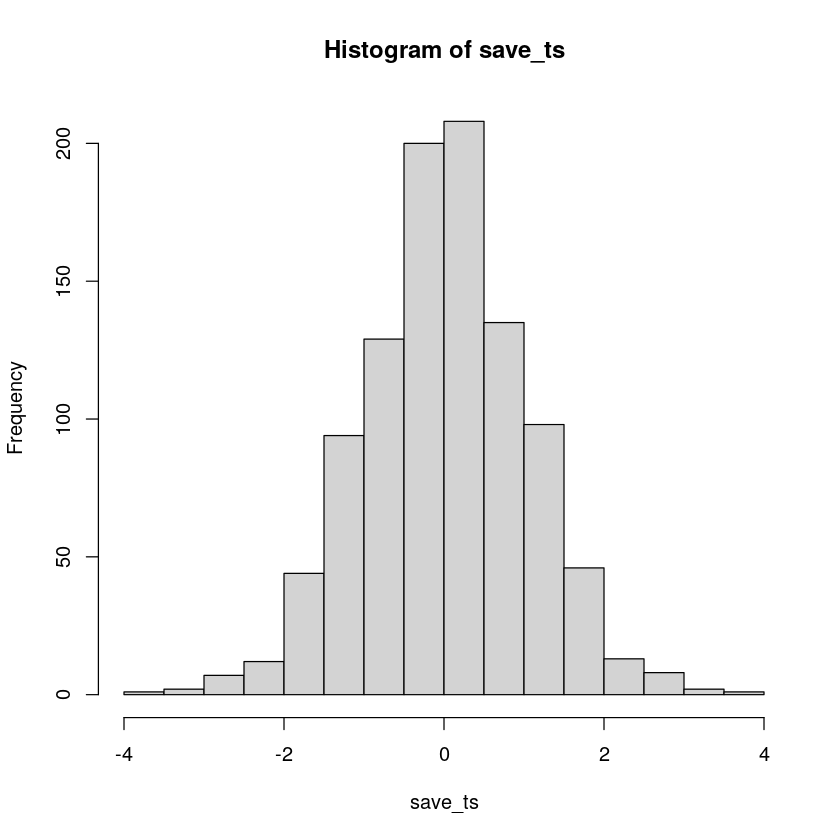Figure $$\PageIndex{1}$$: The distribution of p-values is flat under the null.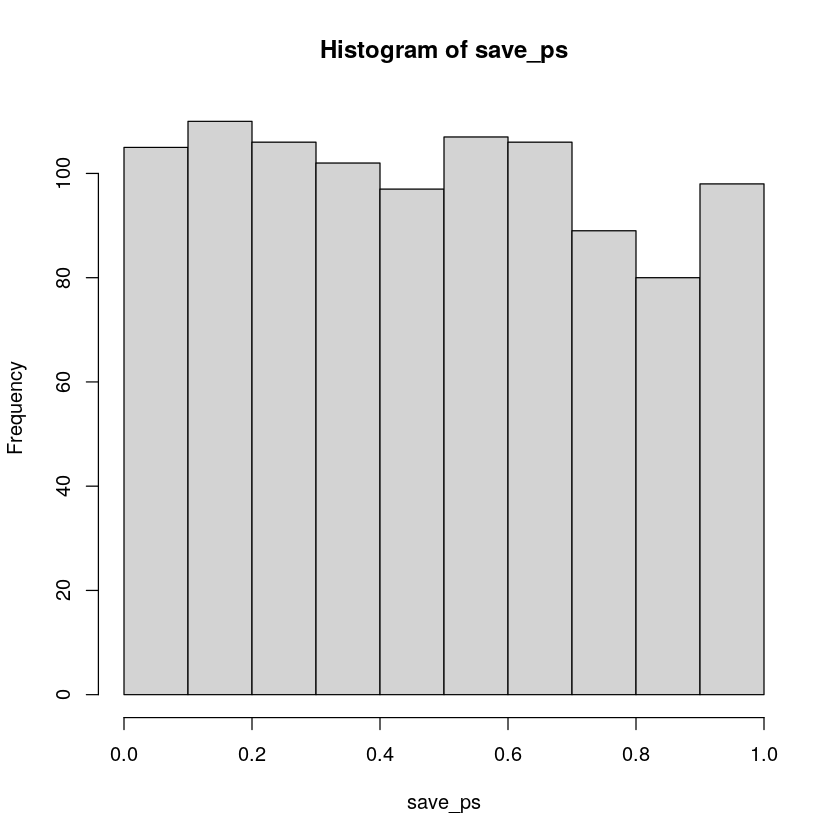Figure $$\PageIndex{2}$$: The distribution of p-values is flat under the null.

Neat. We see both a $$t$$ distribution, that looks like $$t$$ distribution as it should. And we see the $$p$$ distribution. This shows us how often we get $$t$$ values of particular sizes. You may find it interesting that the $$p$$-distribution is flat under the null, which we are simulating here. This means that you have the same chances of a getting a $$t$$ with a p-value between 0 and 0.05, as you would for getting a $$t$$ with a p-value between .90 and .95. Those ranges are both ranges of 5%, so there are an equal amount of $$t$$ values in them by definition.

Here’s another way to do the same simulation in R, using the replicate function, instead a for loop: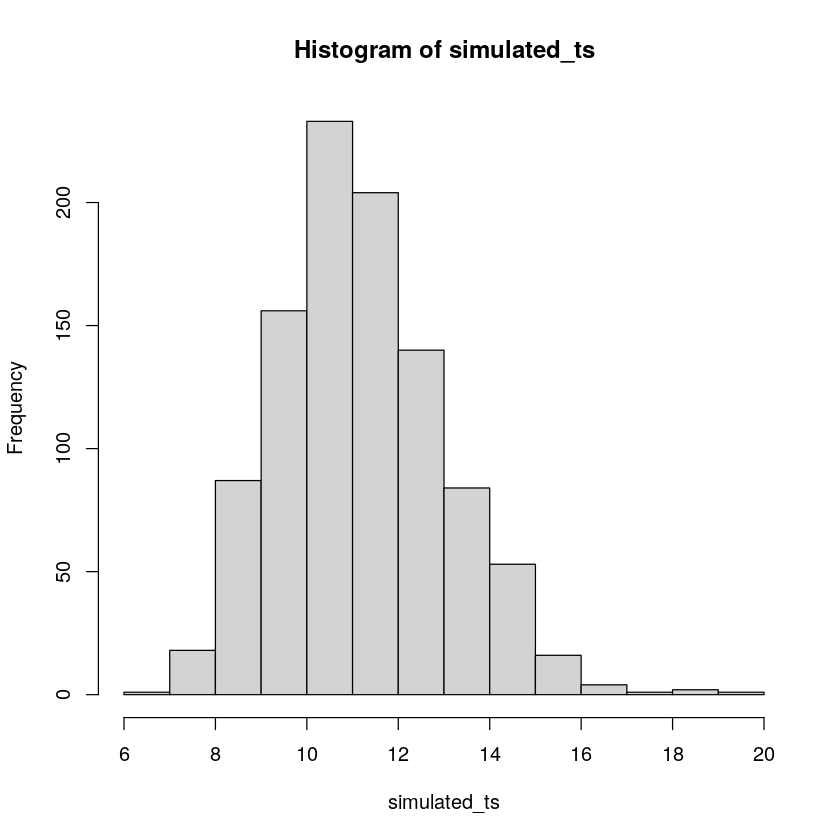Figure $$\PageIndex{3}$$: Simulating ts in R.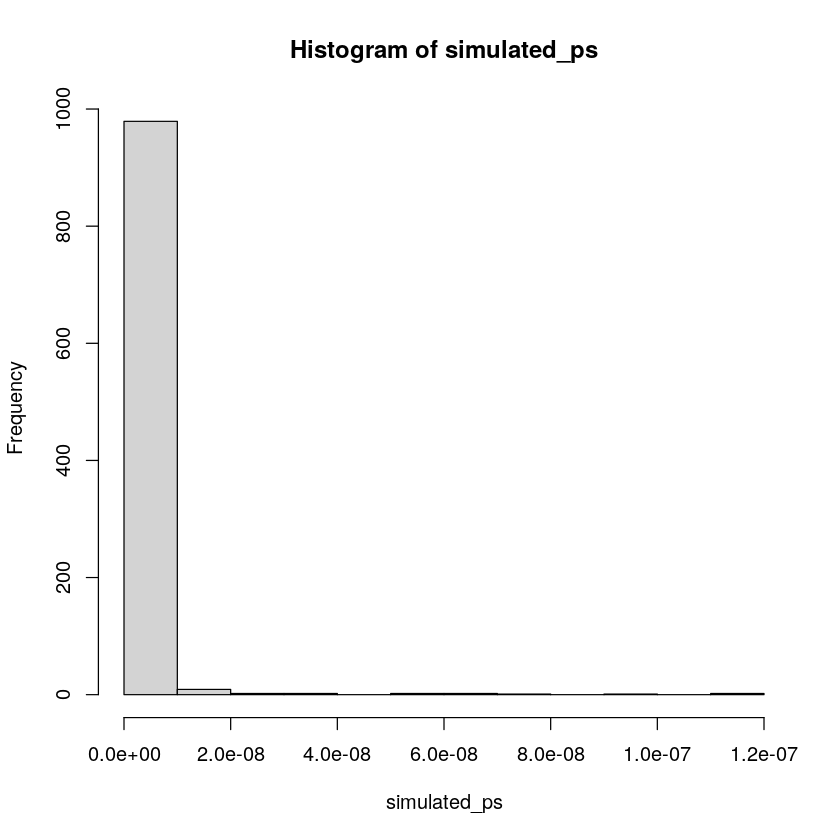Figure $$\PageIndex{4}$$: Simulating ps in R.

## Simulating a paired samples t-test

The code below is set up to sample 10 scores for condition A and B from the same normal distribution. The simulation is conducted 1000 times, and the $$t$$s and $$p$$s are saved and plotted for each.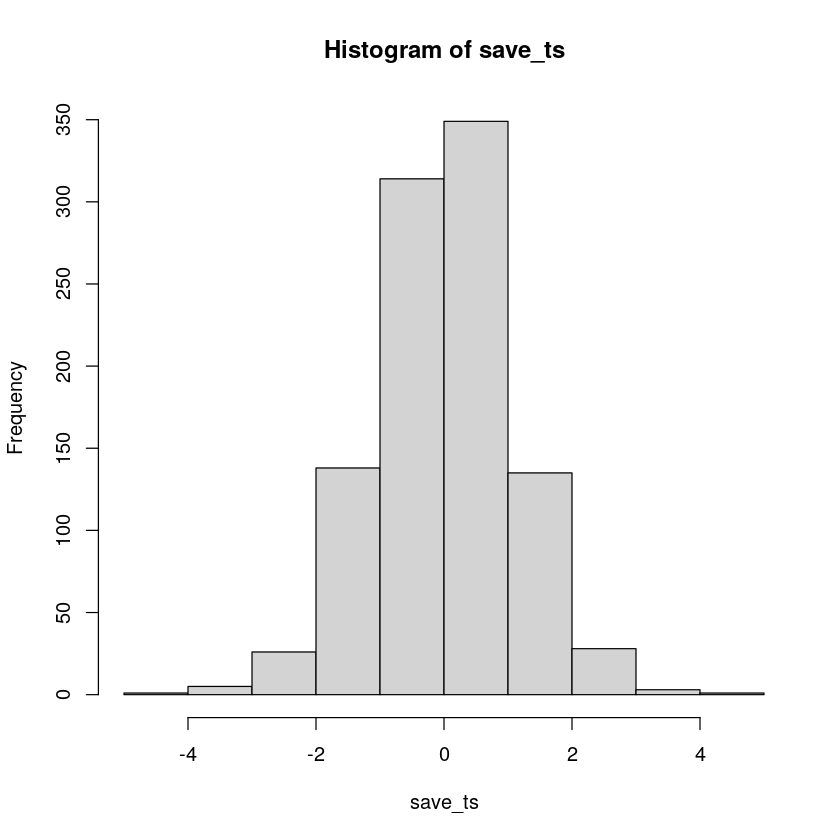Figure $$\PageIndex{5}$$: 1000 simulated ts from the null distribution.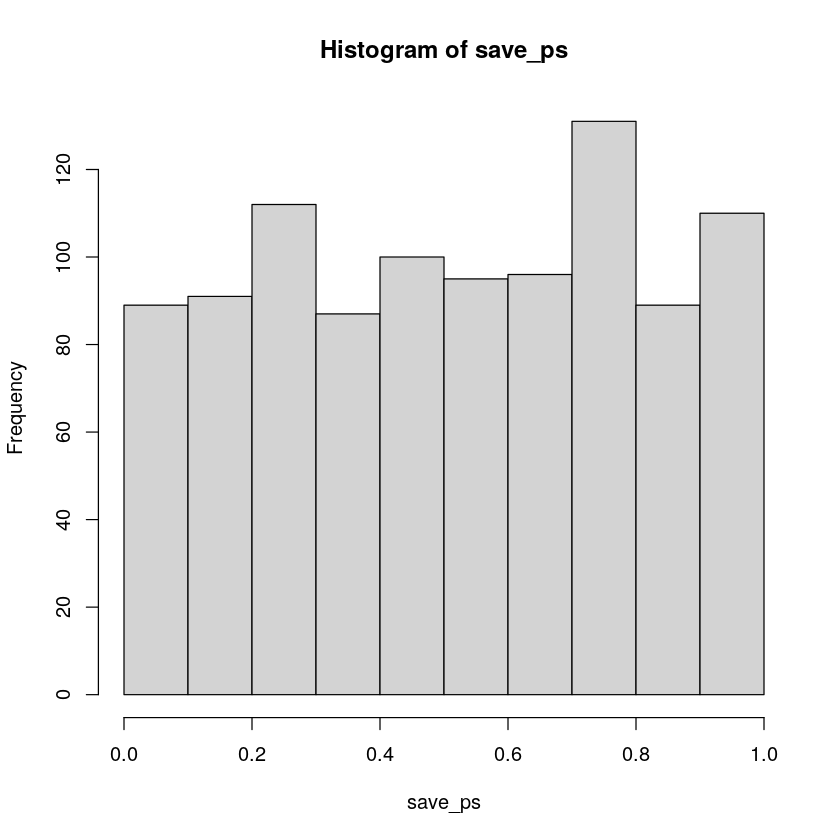Figure $$\PageIndex{6}$$: 1000 simulated ps from the null distribution.

According to the simulation. When there are no differences between the conditions, and the samples are being pulled from the very same distribution, you get these two distributions for $$t$$ and $$p$$. These again show how the null distribution of no differences behaves.

For any of these simulations, if you rejected the null-hypothesis (that your difference was only due to chance), you would be making a type I error. If you set your alpha criteria to $$\alpha = .05$$, we can ask how many type I errors were made in these 1000 simulations. The answer is:

save_ps <- length(1000)
save_ts <- length(1000)
for ( i in 1:1000 ){
condition_A <- rnorm(10,10,5)
condition_B <- rnorm(10,10,5)
differences <- condition_A - condition_B
t_test <- t.test(differences, mu=0)
save_ps[i] <- t_test$p.value save_ts[i] <- t_test$statistic
}
length(save_ps[save_ps<.05])
58
save_ps <- length(1000)
save_ts <- length(1000)
for ( i in 1:1000 ){
condition_A <- rnorm(10,10,5)
condition_B <- rnorm(10,10,5)
differences <- condition_A - condition_B
t_test <- t.test(differences, mu=0)
save_ps[i] <- t_test$p.value save_ts[i] <- t_test$statistic
}
length(save_ps[save_ps<.05])/1000
0.054

We happened to make 55. The expectation over the long run is 5% type I error rates (if your alpha is .05).

What happens if there actually is a difference in the simulated data, let’s set one condition to have a larger mean than the other: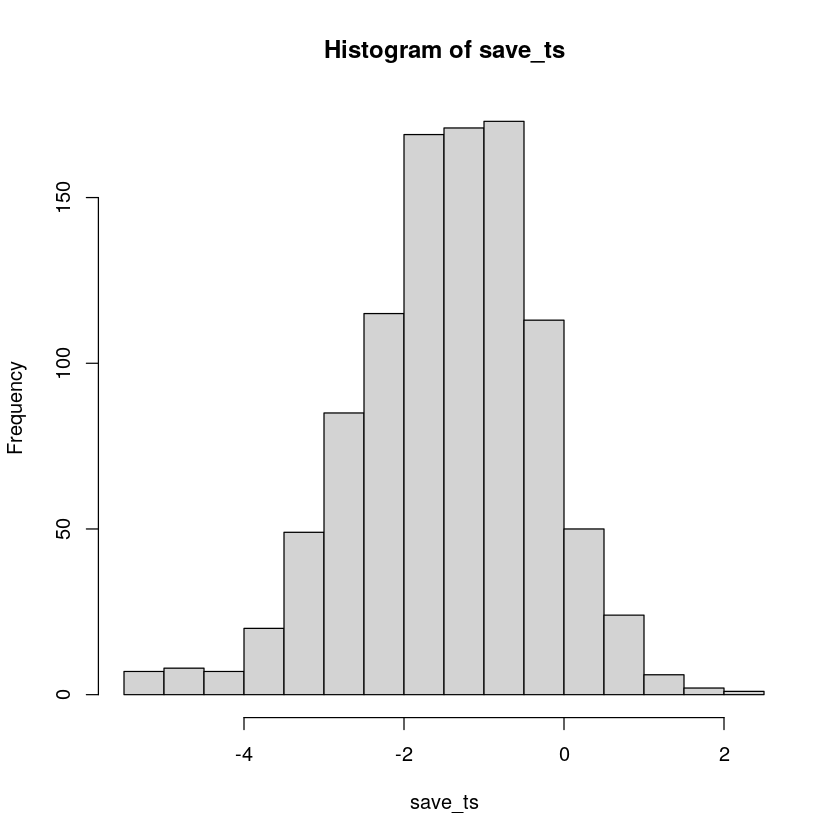Figure $$\PageIndex{7}$$: 1000 ts when there is a true difference.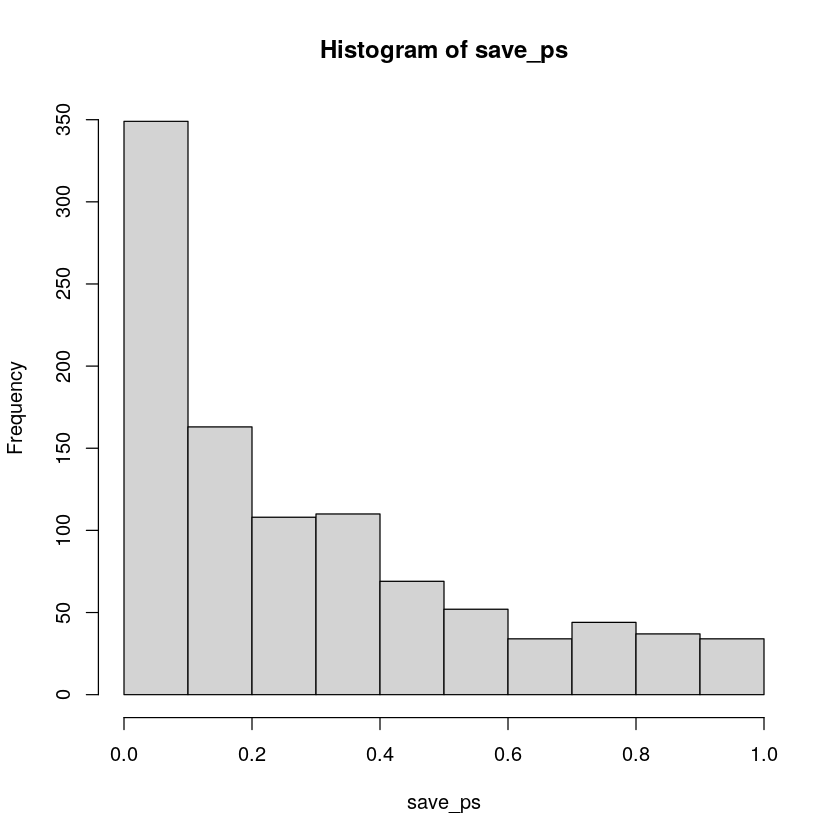Figure $$\PageIndex{8}$$: 1000 ps when there is a true difference.

Now you can see that the $$p$$-value distribution is skewed to the left. This is because when there is a true effect, you will get p-values that are less than .05 more often. Or, rather, you get larger $$t$$ values than you normally would if there were no differences.

In this case, we wouldn’t be making a type I error if we rejected the null when p was smaller than .05. How many times would we do that out of our 1000 experiments?

save_ps <- length(1000)
save_ts <- length(1000)
for ( i in 1:1000 ){
condition_A <- rnorm(10,10,5)
condition_B <- rnorm(10,13,5)
differences <- condition_A - condition_B
t_test <- t.test(differences, mu=0)
save_ps[i] <- t_test$p.value save_ts[i] <- t_test$statistic
}
length(save_ps[save_ps<.05])
210
save_ps <- length(1000)
save_ts <- length(1000)
for ( i in 1:1000 ){
condition_A <- rnorm(10,10,5)
condition_B <- rnorm(10,13,5)
differences <- condition_A - condition_B
t_test <- t.test(differences, mu=0)
save_ps[i] <- t_test$p.value save_ts[i] <- t_test$statistic
}
length(save_ps[save_ps<.05])/1000
0.21

We happened to get 210 simulations where p was less than .05, that’s only 0.21 experiments. If you were the researcher, would you want to run an experiment that would be successful only 0.21 of the time? I wouldn’t. I would run a better experiment.

How would you run a better simulated experiment? Well, you could increase $$n$$, the number of subjects in the experiment. Let’s increase $$n$$ from 10 to 100, and see what happens to the number of “significant” simulated experiments.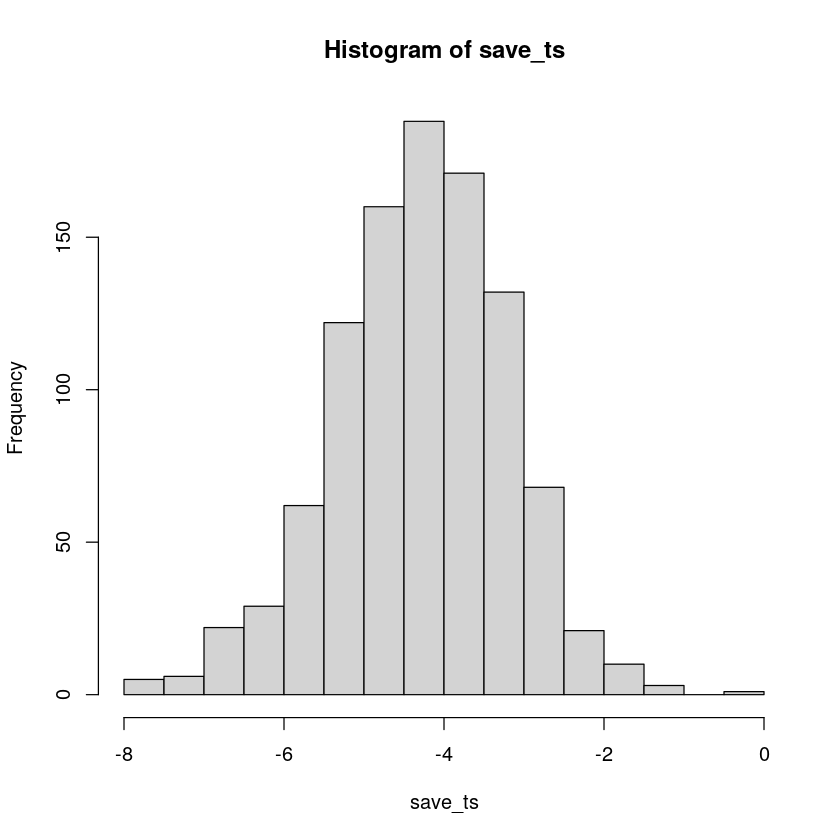Figure $$\PageIndex{9}$$: 1000 ts for n =100, when there is a true effect.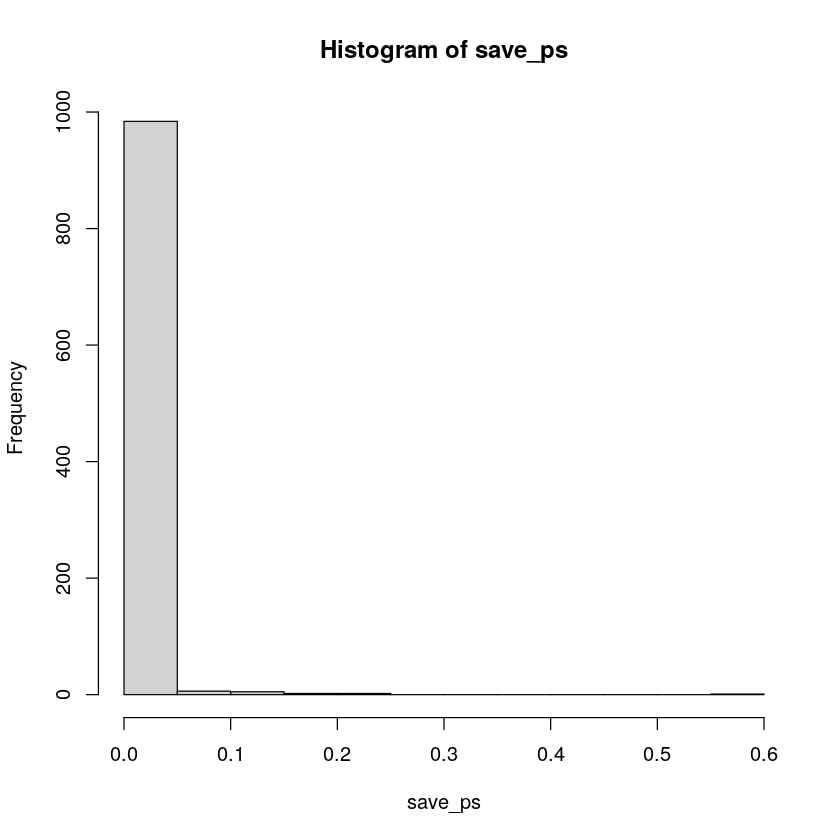Figure $$\PageIndex{10}$$: 1000 ps for n =100, when there is a true effect.
save_ps <- length(1000)
save_ts <- length(1000)
for ( i in 1:1000 ){
condition_A <- rnorm(100,10,5)
condition_B <- rnorm(100,13,5)
differences <- condition_A - condition_B
t_test <- t.test(differences, mu=0)
save_ps[i] <- t_test$p.value save_ts[i] <- t_test$statistic
}
length(save_ps[save_ps<.05])
985
save_ps <- length(1000)
save_ts <- length(1000)
for ( i in 1:1000 ){
condition_A <- rnorm(100,10,5)
condition_B <- rnorm(100,13,5)
differences <- condition_A - condition_B
t_test <- t.test(differences, mu=0)
save_ps[i] <- t_test$p.value save_ts[i] <- t_test$statistic
}
length(save_ps[save_ps<.05])/1000
0.985

Cool, now almost all of the experiments show a $$p$$-value of less than .05 (using a two-tailed test, that’s the default in R). See, you could use this simulation process to determine how many subjects you need to reliably find your effect.

## Simulating an independent samples t.test

Just change the t.test function like so… this is for the null, assuming no difference between groups.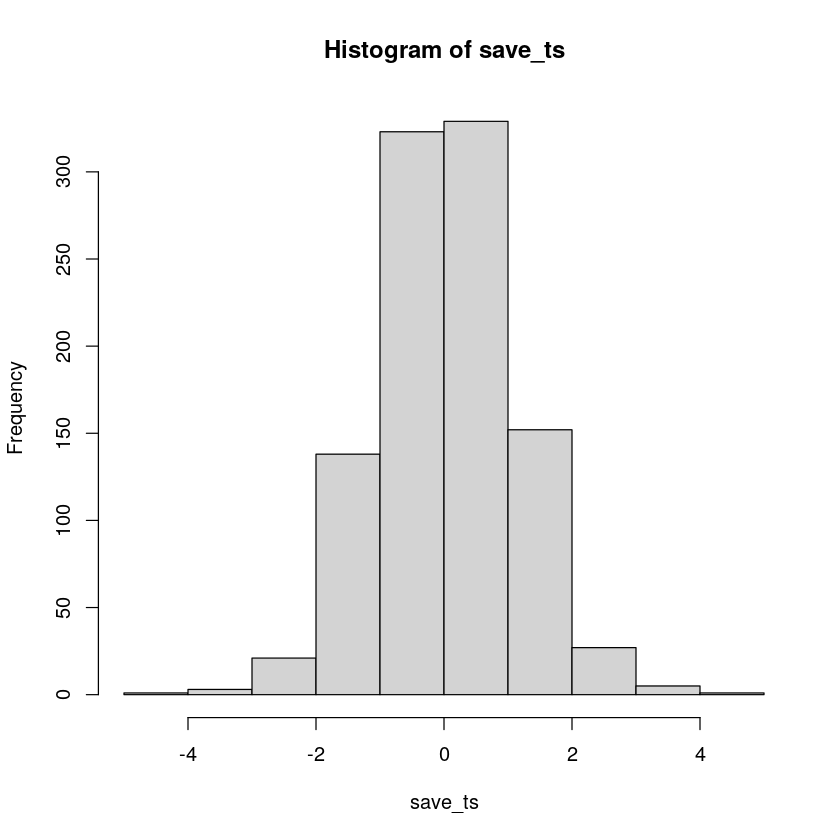Figure $$\PageIndex{11}$$: 1000 ts for n =100, when there is a true effect.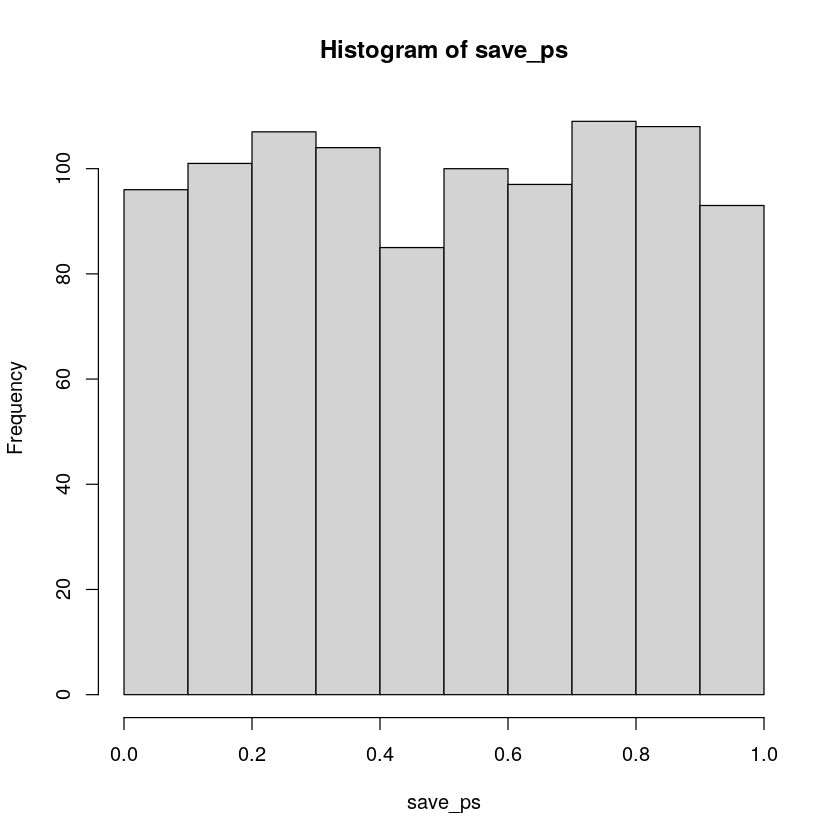Figure $$\PageIndex{12}$$: 1000 ps for n =100, when there is a true effect.
save_ps <- length(1000)
save_ts <- length(1000)
for ( i in 1:1000 ){
group_A <- rnorm(10,10,5)
group_B <- rnorm(10,10,5)
t_test <- t.test(group_A, group_B, paired=FALSE, var.equal=TRUE)
save_ps[i] <- t_test$p.value save_ts[i] <- t_test$statistic
}
length(save_ps[save_ps<.05])
41
save_ps <- length(1000)
save_ts <- length(1000)
for ( i in 1:1000 ){
group_A <- rnorm(10,10,5)
group_B <- rnorm(10,10,5)
t_test <- t.test(group_A, group_B, paired=FALSE, var.equal=TRUE)
save_ps[i] <- t_test$p.value save_ts[i] <- t_test$statistic
}
length(save_ps[save_ps<.05])/1000
0.041

This page titled 6.6: Simulating data for t-tests is shared under a CC BY-SA 4.0 license and was authored, remixed, and/or curated by Matthew J. C. Crump via source content that was edited to the style and standards of the LibreTexts platform; a detailed edit history is available upon request.